Скачать презентацию Chapter 2 Functions and Graphs Section 6 Logarithmic

e06f602aa5e5e3ba47ede3e934cb223f.ppt

• Количество слайдов: 27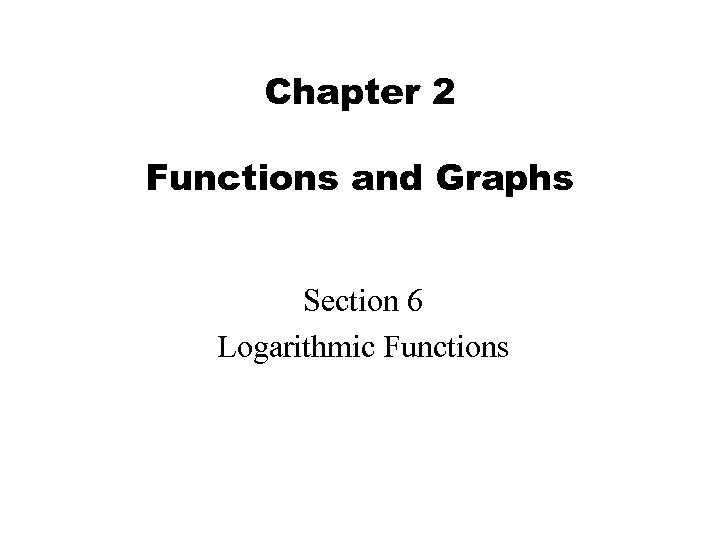Chapter 2 Functions and Graphs Section 6 Logarithmic Functions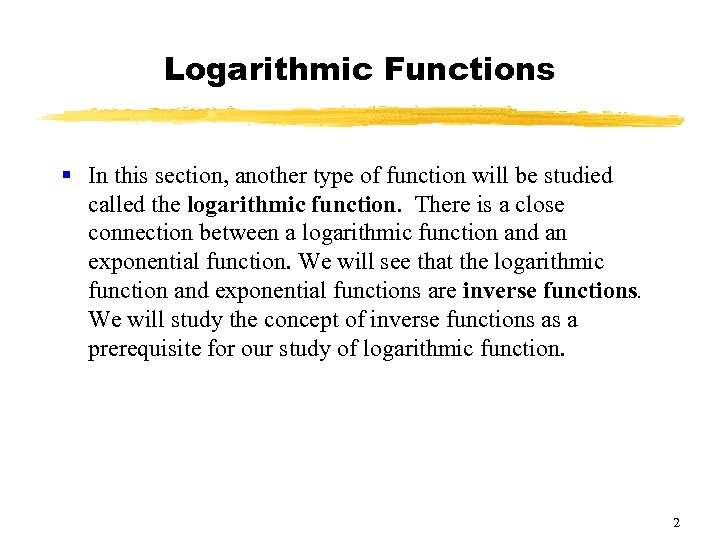Logarithmic Functions § In this section, another type of function will be studied called the logarithmic function. There is a close connection between a logarithmic function and an exponential function. We will see that the logarithmic function and exponential functions are inverse functions. We will study the concept of inverse functions as a prerequisite for our study of logarithmic function. 2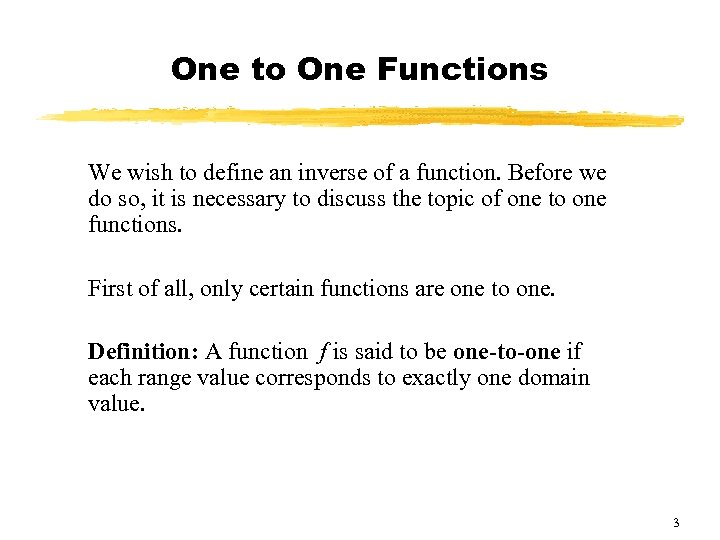One to One Functions We wish to define an inverse of a function. Before we do so, it is necessary to discuss the topic of one to one functions. First of all, only certain functions are one to one. Definition: A function f is said to be one-to-one if each range value corresponds to exactly one domain value. 3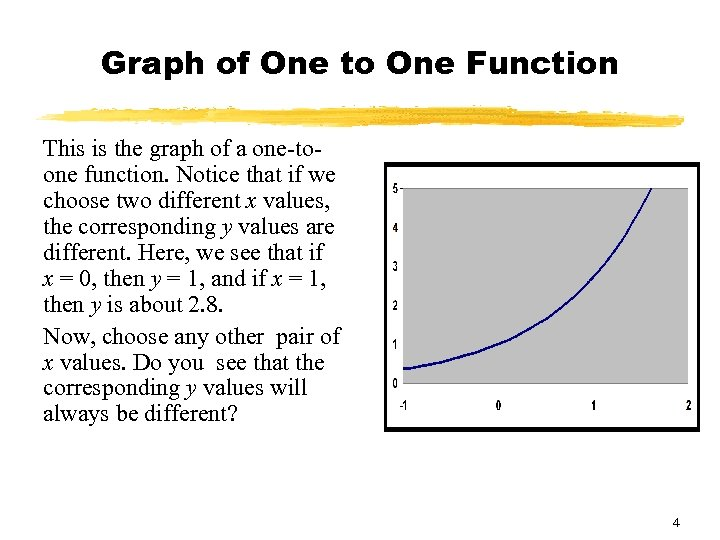Graph of One to One Function This is the graph of a one-toone function. Notice that if we choose two different x values, the corresponding y values are different. Here, we see that if x = 0, then y = 1, and if x = 1, then y is about 2. 8. Now, choose any other pair of x values. Do you see that the corresponding y values will always be different? 4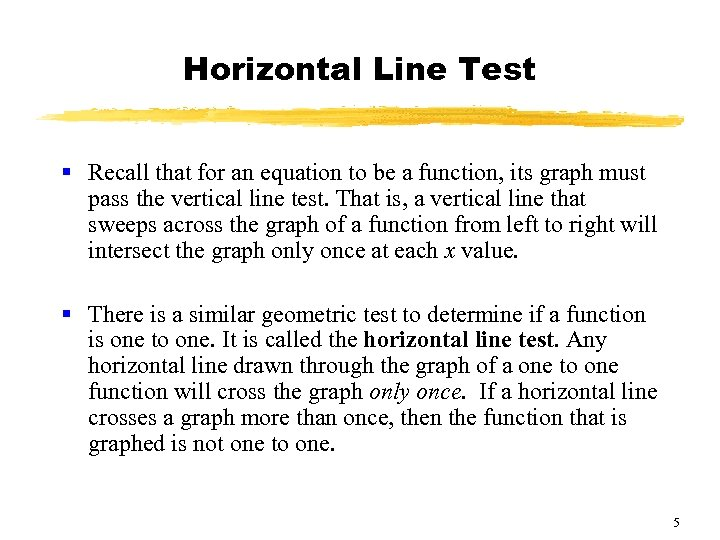Horizontal Line Test § Recall that for an equation to be a function, its graph must pass the vertical line test. That is, a vertical line that sweeps across the graph of a function from left to right will intersect the graph only once at each x value. § There is a similar geometric test to determine if a function is one to one. It is called the horizontal line test. Any horizontal line drawn through the graph of a one to one function will cross the graph only once. If a horizontal line crosses a graph more than once, then the function that is graphed is not one to one. 5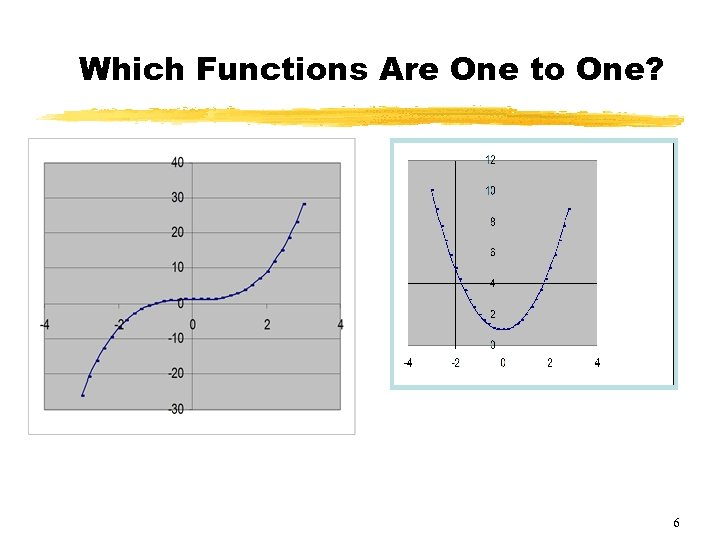Which Functions Are One to One? 6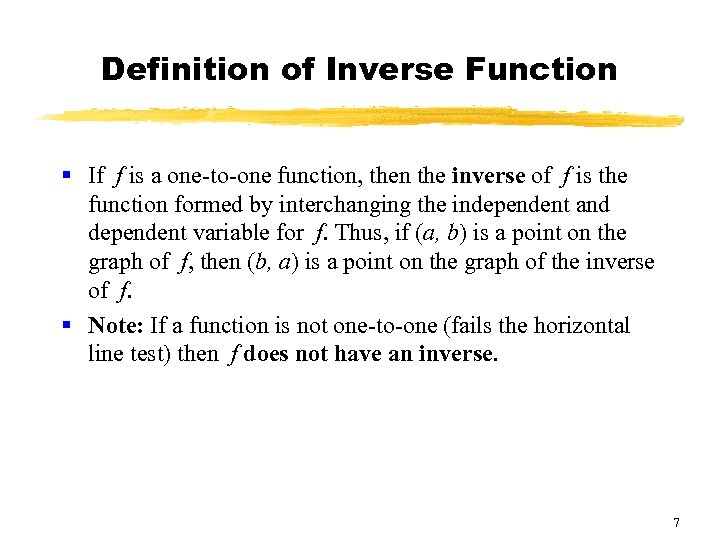Definition of Inverse Function § If f is a one-to-one function, then the inverse of f is the function formed by interchanging the independent and dependent variable for f. Thus, if (a, b) is a point on the graph of f, then (b, a) is a point on the graph of the inverse of f. § Note: If a function is not one-to-one (fails the horizontal line test) then f does not have an inverse. 7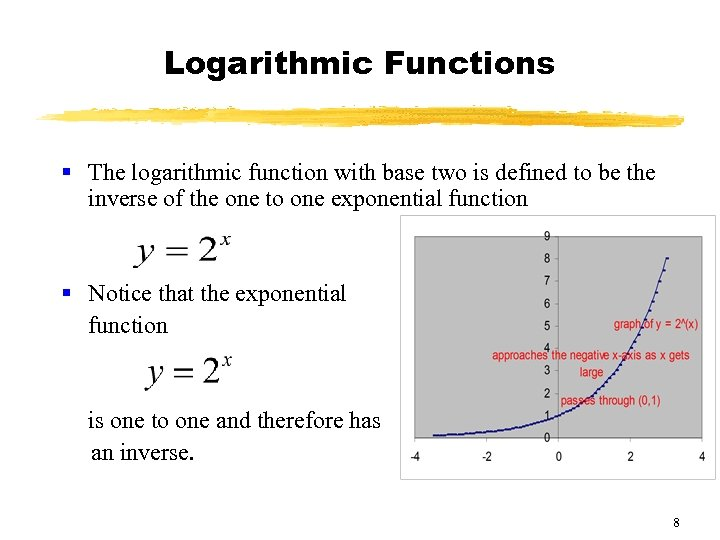Logarithmic Functions § The logarithmic function with base two is defined to be the inverse of the one to one exponential function § Notice that the exponential function is one to one and therefore has an inverse. 8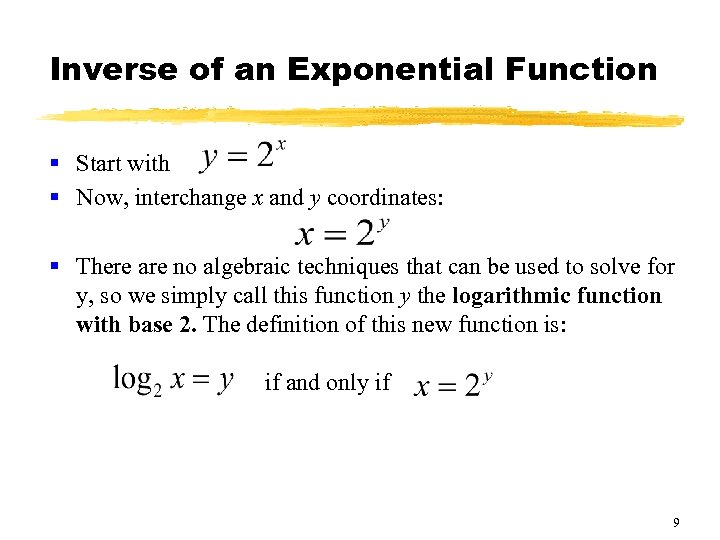Inverse of an Exponential Function § Start with § Now, interchange x and y coordinates: § There are no algebraic techniques that can be used to solve for y, so we simply call this function y the logarithmic function with base 2. The definition of this new function is: if and only if 9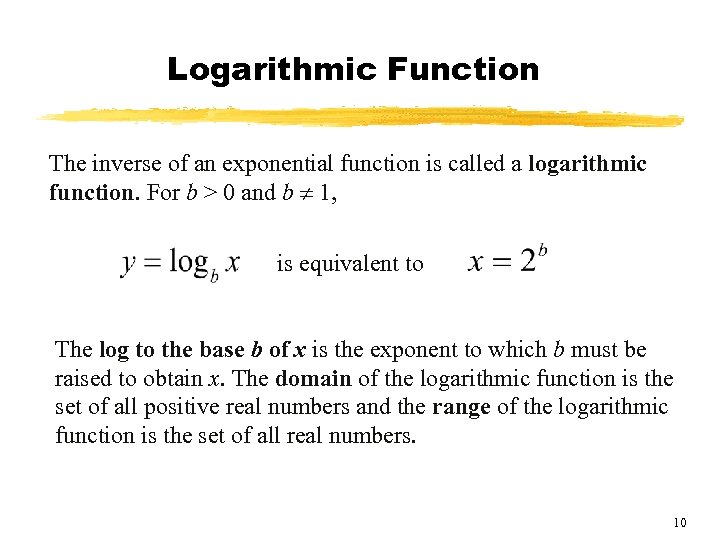Logarithmic Function The inverse of an exponential function is called a logarithmic function. For b > 0 and b 1, is equivalent to The log to the base b of x is the exponent to which b must be raised to obtain x. The domain of the logarithmic function is the set of all positive real numbers and the range of the logarithmic function is the set of all real numbers. 10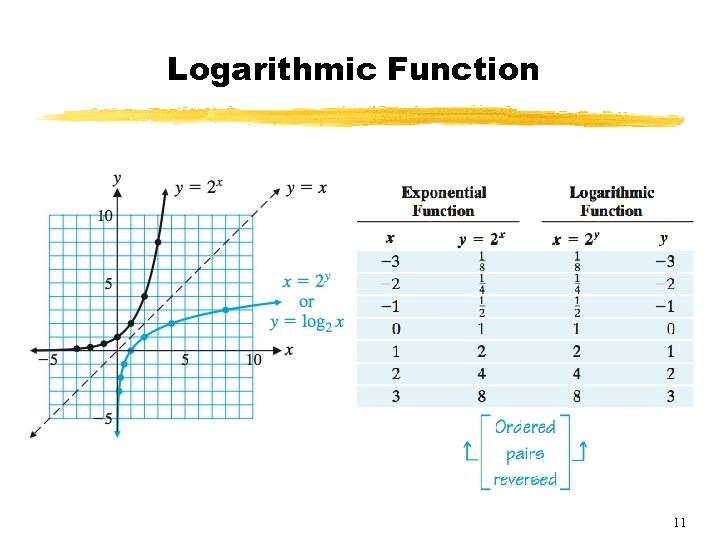Logarithmic Function 11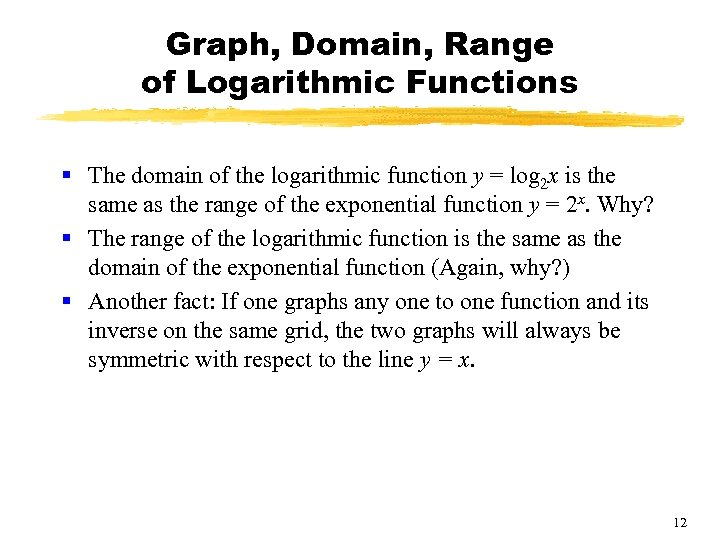Graph, Domain, Range of Logarithmic Functions § The domain of the logarithmic function y = log 2 x is the same as the range of the exponential function y = 2 x. Why? § The range of the logarithmic function is the same as the domain of the exponential function (Again, why? ) § Another fact: If one graphs any one to one function and its inverse on the same grid, the two graphs will always be symmetric with respect to the line y = x. 12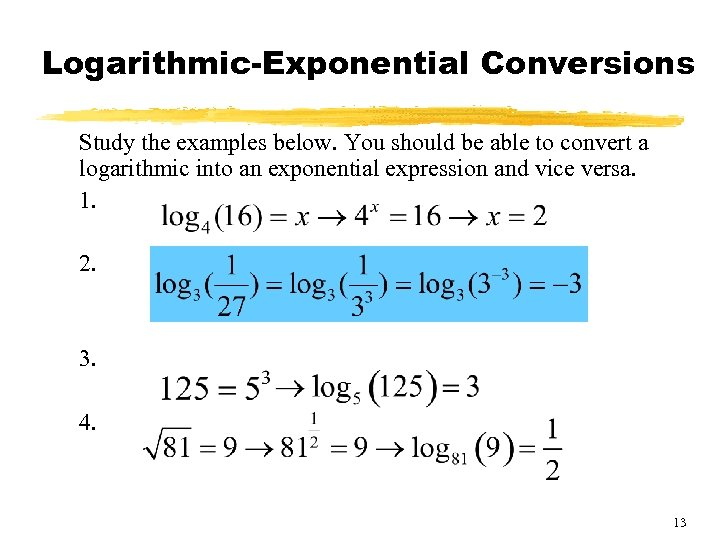Logarithmic-Exponential Conversions Study the examples below. You should be able to convert a logarithmic into an exponential expression and vice versa. 1. 2. 3. 4. 13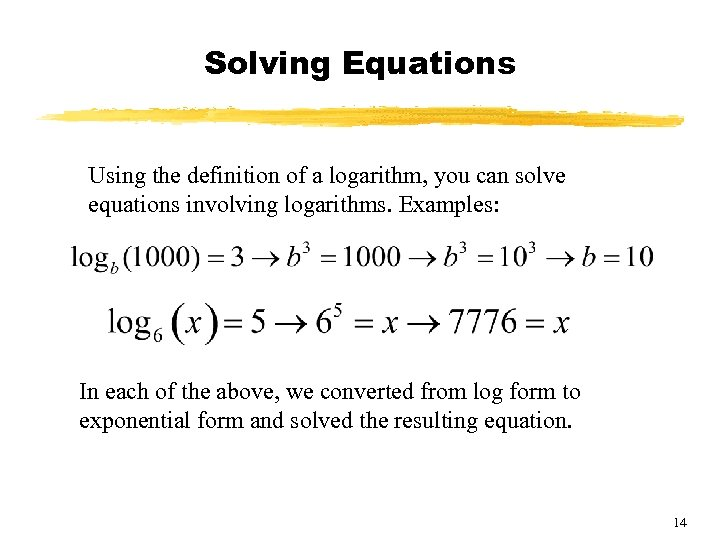Solving Equations Using the definition of a logarithm, you can solve equations involving logarithms. Examples: In each of the above, we converted from log form to exponential form and solved the resulting equation. 14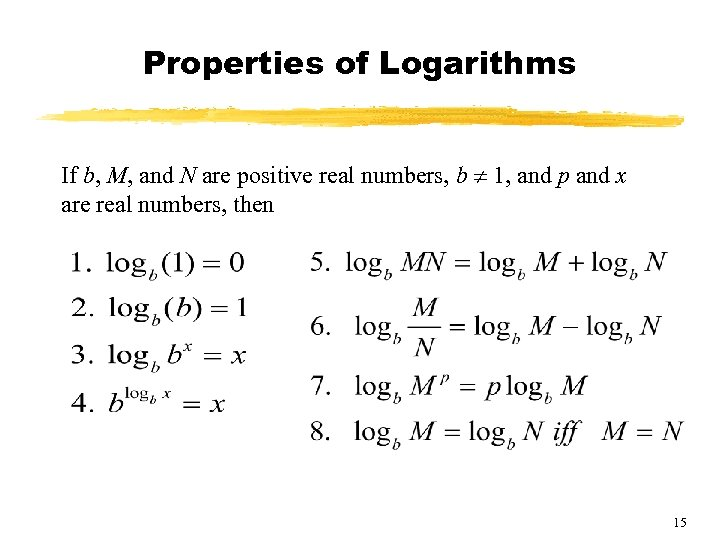Properties of Logarithms If b, M, and N are positive real numbers, b 1, and p and x are real numbers, then 15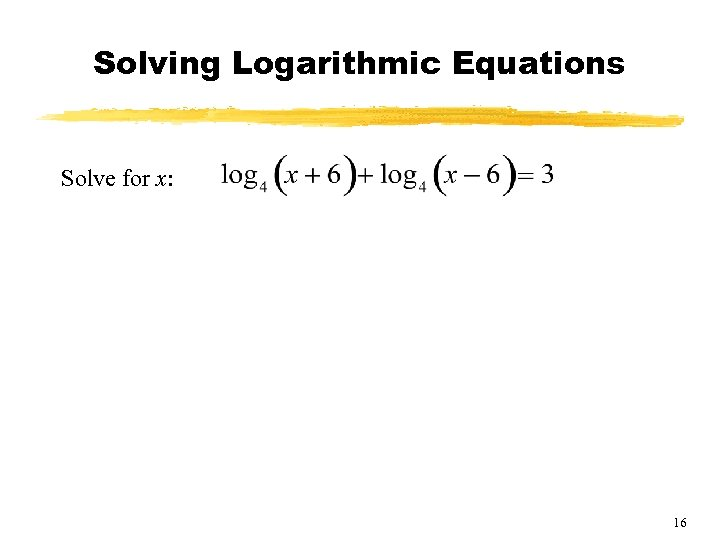Solving Logarithmic Equations Solve for x: 16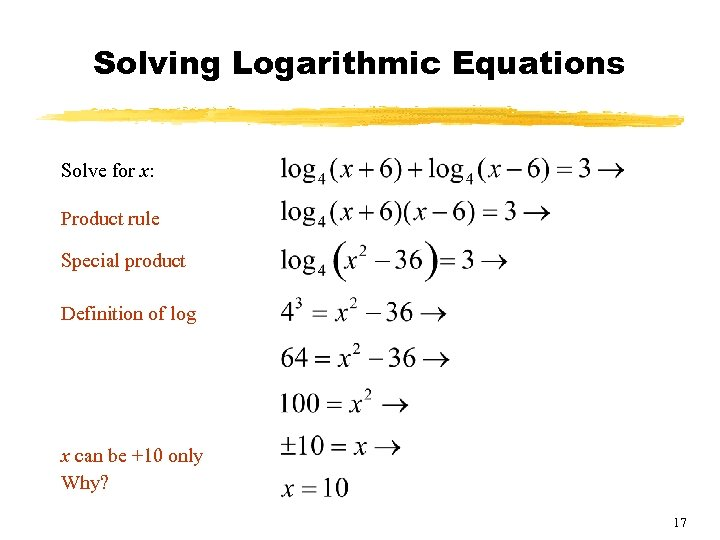Solving Logarithmic Equations Solve for x: Product rule Special product Definition of log x can be +10 only Why? 17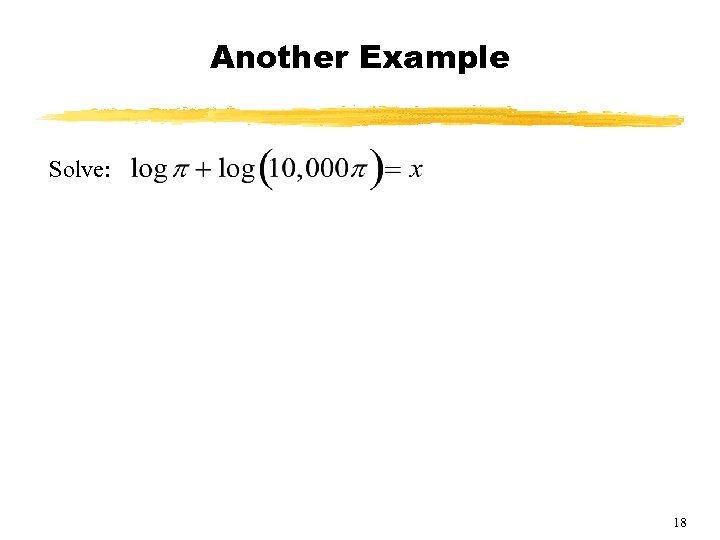Another Example Solve: 18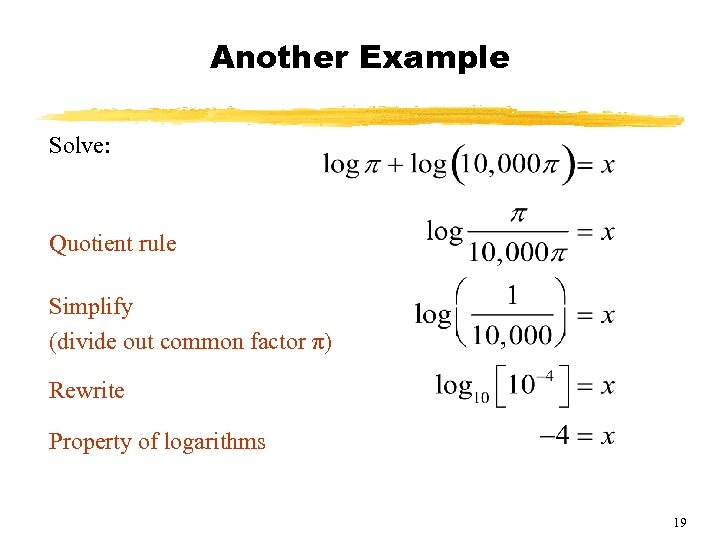Another Example Solve: Quotient rule Simplify (divide out common factor π) Rewrite Property of logarithms 19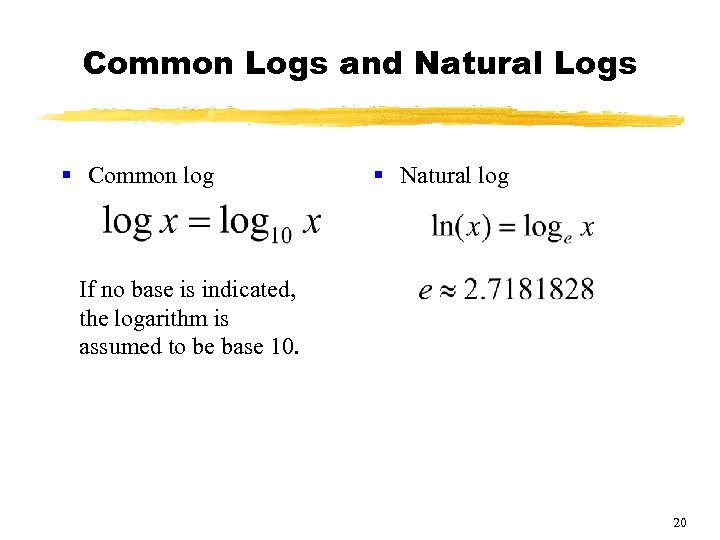Common Logs and Natural Logs § Common log § Natural log If no base is indicated, the logarithm is assumed to be base 10. 20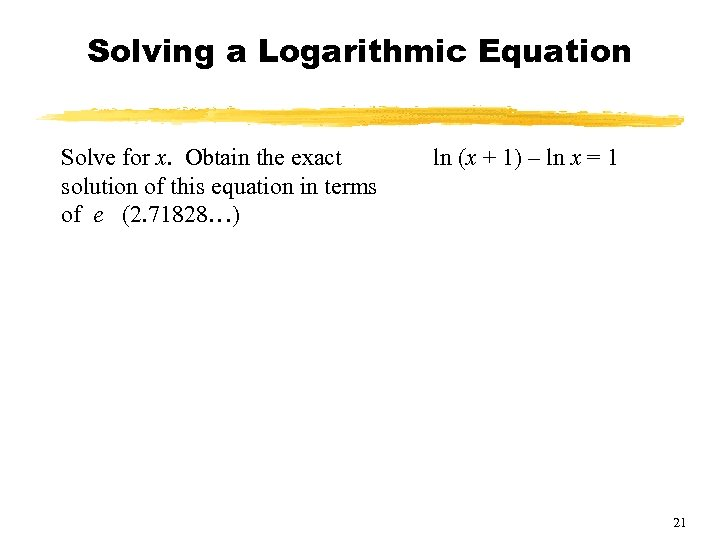Solving a Logarithmic Equation Solve for x. Obtain the exact solution of this equation in terms of e (2. 71828…) ln (x + 1) – ln x = 1 21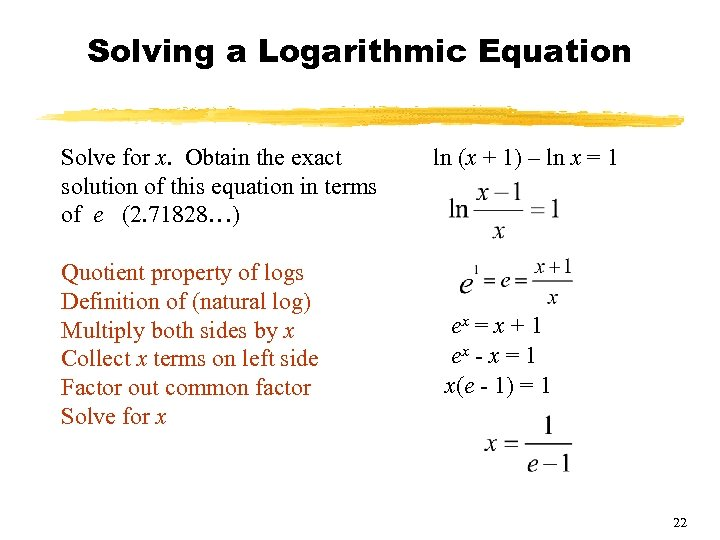Solving a Logarithmic Equation Solve for x. Obtain the exact solution of this equation in terms of e (2. 71828…) Quotient property of logs Definition of (natural log) Multiply both sides by x Collect x terms on left side Factor out common factor Solve for x ln (x + 1) – ln x = 1 ex = x + 1 ex - x = 1 x(e - 1) = 1 22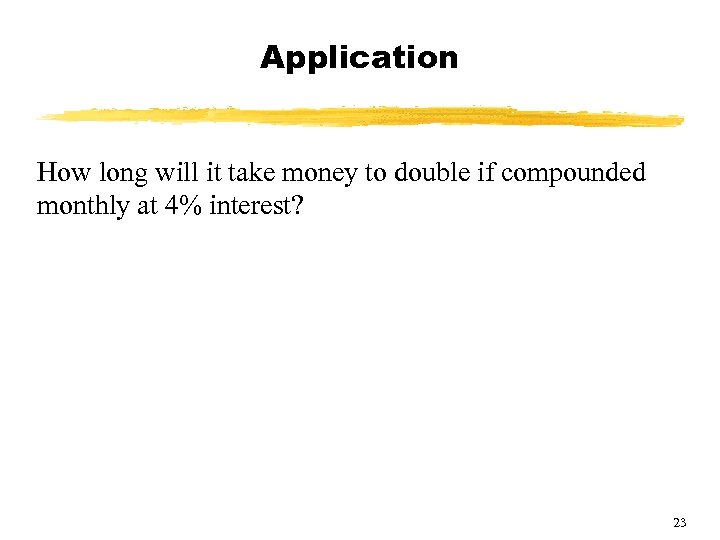Application How long will it take money to double if compounded monthly at 4% interest? 23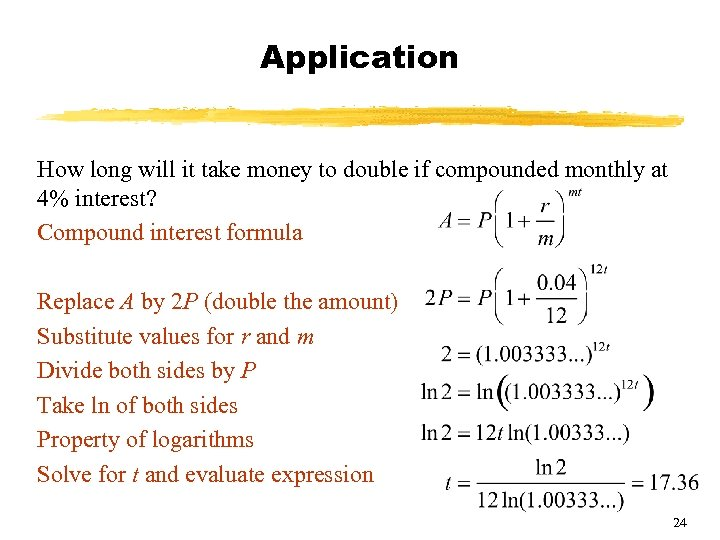Application How long will it take money to double if compounded monthly at 4% interest? Compound interest formula Replace A by 2 P (double the amount) Substitute values for r and m Divide both sides by P Take ln of both sides Property of logarithms Solve for t and evaluate expression 24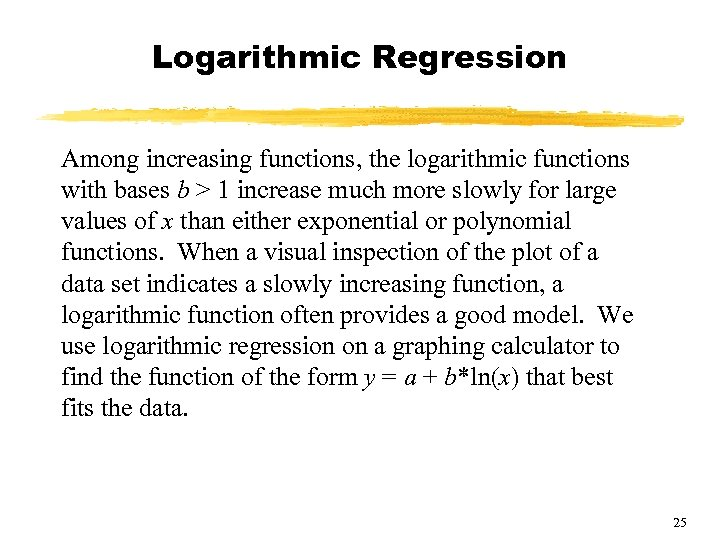Logarithmic Regression Among increasing functions, the logarithmic functions with bases b > 1 increase much more slowly for large values of x than either exponential or polynomial functions. When a visual inspection of the plot of a data set indicates a slowly increasing function, a logarithmic function often provides a good model. We use logarithmic regression on a graphing calculator to find the function of the form y = a + b*ln(x) that best fits the data. 25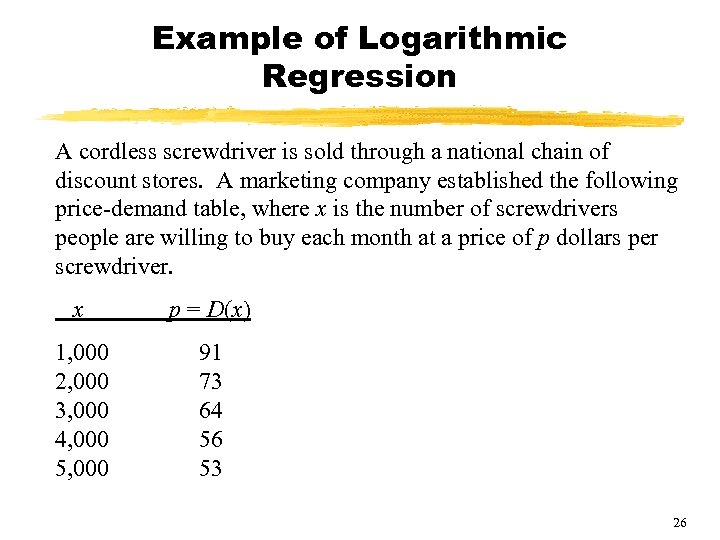Example of Logarithmic Regression A cordless screwdriver is sold through a national chain of discount stores. A marketing company established the following price-demand table, where x is the number of screwdrivers people are willing to buy each month at a price of p dollars per screwdriver. x p = D(x) 1, 000 2, 000 3, 000 4, 000 5, 000 91 73 64 56 53 26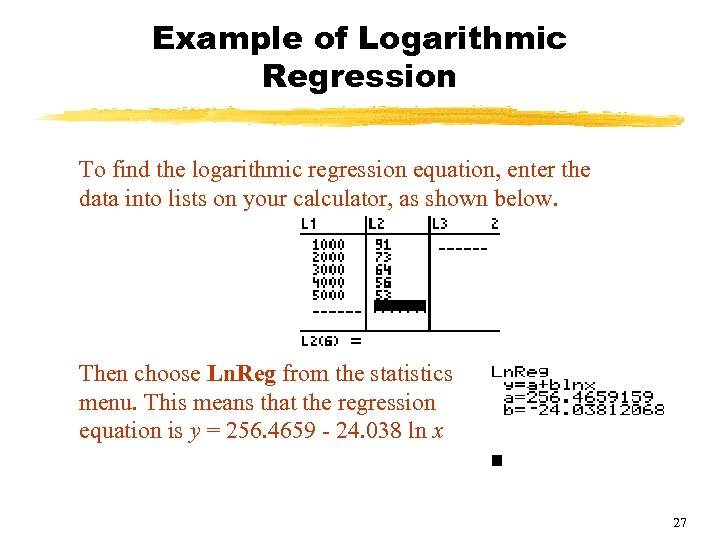Example of Logarithmic Regression To find the logarithmic regression equation, enter the data into lists on your calculator, as shown below. Then choose Ln. Reg from the statistics menu. This means that the regression equation is y = 256. 4659 - 24. 038 ln x 27